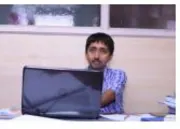X
Study of memory effect in an inventory model for deteriorating items with partial backlogging
R. Pakhira,
Published in Elsevier Ltd
2020
Volume: 148

Abstract
A generalization of the economic order quantity (EOQ) model is taken into account to study the memory effect in the inventory model. Fractional calculus is one of the efficient medium to establish the existence of memory effect in the economic system. This paper deals with a system of two fractional order differential equations to govern the fractional order EOQ model with partial backlogging. The fractional order differential equation is considered here in Caputo sense. The theory of memory kernel is applied to set up the memory dependent EOQ model. Using primal geometric programming method, analytical solution of the proposed problem is found. Using empirical values of the system parameters, we have established the concept of short and long memory effect. It is clear from the investigations that the strength of memory can be controlled by the order of fractional derivative or integration. Sensitivity analysis has been carried out for long memory and short memory affected problem both to identify the important model parameters for different situations. Finally, the manuscript is concluded with some recommendation about the memory dependent EOQ model. © 2020 Elsevier Ltd
••